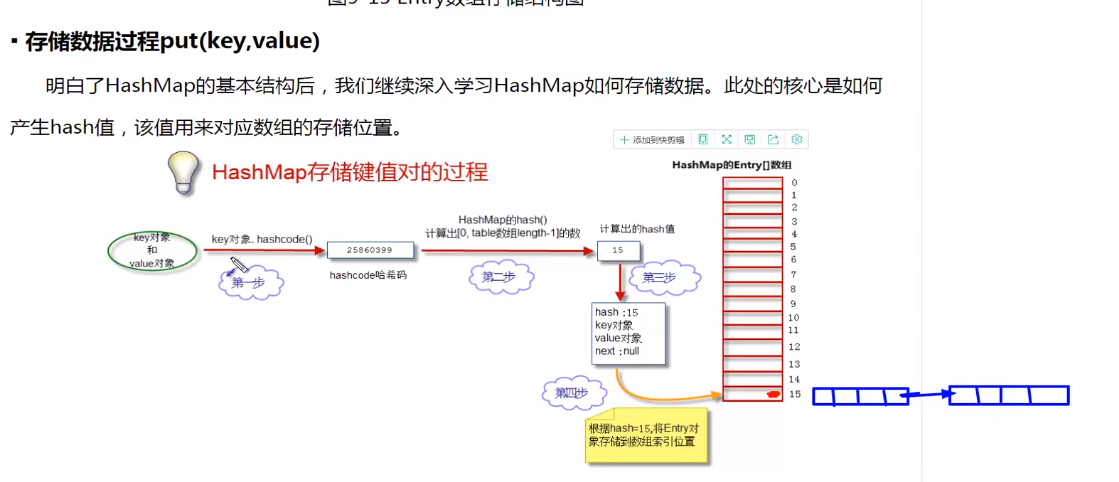# 二、jdk1.8

final V putVal(int hash, K key, V value, boolean onlyIfAbsent,
2 boolean evict) {
3 Node<K,V>[] tab; Node<K,V> p; int n, i;
4 if ((tab = table) == null || (n = tab.length) == 0)
5 n = (tab = resize()).length;
6 if ((p = tab[i = (n – 1) & hash]) == null) // 如果没有hash碰撞则直接插入元素
7 tab[i] = newNode(hash, key, value, null);
8 else {
9 Node<K,V> e; K k;
10 if (p.hash == hash &&
11 ((k = p.key) == key || (key != null && key.equals(k))))
12 e = p;
13 else if (p instanceof TreeNode)
14 e = ((TreeNode<K,V>)p).putTreeVal(this, tab, hash, key, value);
15 else {
16 for (int binCount = 0; ; ++binCount) {
17 if ((e = p.next) == null) {
18 p.next = newNode(hash, key, value, null);
19 if (binCount >= TREEIFY_THRESHOLD – 1) // -1 for 1st
20 treeifyBin(tab, hash);
21 break;
22 }
23 if (e.hash == hash &&
24 ((k = e.key) == key || (key != null && key.equals(k))))
25 break;
26 p = e;
27 }
28 }
29 if (e != null) { // existing mapping for key
30 V oldValue = e.value;
31 if (!onlyIfAbsent || oldValue == null)
32 e.value = value;
33 afterNodeAccess(e);
34 return oldValue;
35 }
36 }
37 ++modCount;
38 if (++size > threshold)
39 resize();
40 afterNodeInsertion(evict);
41 return null;
42 }

### #2.在jdk1.8中，在多线程环境下，会发生数据覆盖的情况。